Personal Study Notes: Java Programming

Java's primitive types, character and numeric literals. How to declare vari­ables, arrays of variables, and arrays of objects. How to initialise variables. Java expressions and type conversions. How to access members of a class, including overloaded methods. Integer and floating-point arithmetic. Java's ++, relational, bitwise, conditional, assignment operators and their preced­ence. Java's flow control

Java's Primitive Types

boolean either true or false 16-bit Unicode 1.1.5 character 8-bit signed two's-complement integer 16-bit signed two's-complement integer 32-bit signed two's-complement integer 64-bit signed two's-complement integer 32-bit IEEE 754-1985 floating-point number 64-bit IEEE 754-1985 floating-point number

short and byte are always promoted to int before being evaluated. They are only stored, never operated upon.

MIN_VALUE = minimum value pre-defined for the type concerned
MAX_VALUE = maximum value pre-defined for the type concerned
float and double can also have stored values of:
• NEGATIVE_INFINITY
• POSITIVE_INFINITY

NaN means 'not a number'. It indicates that the number is an invalid result such as the result of dividing by zero. This can be tested for by the method (function) isNan().

Java's Numeric Literals

null an as yet uncreated, or an invalid, Object Reference the two possible values of a boolean variable int constant - decimal representation int constant - octal (base 8) representation int constant - hexadecimal (base 16) representation

When an int constant (as above) is assigned to a byte variable or a short vari­able, it is automatically converted to byte or short as appropriate (provided its value lies within the valid range for that type).

29L     long constant - decimal representation
035L    long constant - octal (base 8) representation
0x1DL   long constant - hexadecimal (base 16) representation

18.0F   float constant - the .0 is optional, the F is mandatory
1.8E1F  float constant - exponent form, the F is mandatory
.18E2F  float constant - exponent form, the F is mandatory

18.0D   double constant - the .0 and the D are optional
1.8E1D  double constant - exponent form the D is optional
.18E2D  double constant - exponent form the D is optional

Zero can be positive or negative. These are numerically equal but give different results in certain calculations eg: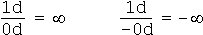A non-float constant such as a double cannot be assigned to a float variable. You must use a float literal viz:

float x = 18.9F;

Java's Character Literals

Character literals appear between single quote marks viz:

char c = 'a';  //make character variable c contain the letter 'a'

Certain special characters are represented by the following escape sequences (which are also placed between single quote marks in assignment statements).

\n \t \u000A new-line \u0009 tab \u0008 back-space \u000D return \u000C form-feed \u005C back-slash \u0027 single quote \u0022 double quote d = 0 to 7 character's octal value d = 0 to F character's hexadecimal value

STRING LITERALS

String literals appear between double quote marks viz:

String s = "help";  /* make string variable s point to a string
array containing the letters 'help' */

To break a line within a quoted string, you must insert a \n into the string, not simply start a new line in your source file. Be careful of how you place octal character entities in strings. For instance:

\0116 is rendered as \t6 (ie a tab followed by a character 6)
\116 is rendered as N.

How to Declare Variables

Access Modifiers

private variable is visible only within the immediate class in which it is declared. variable is visible from anywhere from which the class within which it is declared is visible. variable is visible to classes which extend the class in which it is declared.

Other Modifiers

static variable retains its value after the method in which it is declared terminates. variable is locked from access by other program threads while a particular thread is updating it. variable can only be assigned a value once, that is, when it is initialised just before the first pass of the method code.

The order in which the modifiers are placed in the declaration of a variable are, by convention: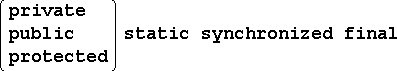The form of a complete variable-declaration is therefore as follows: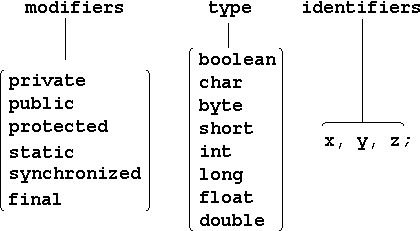The following statement declares three interger variables x, y, z:

private static final int x, y, z;

Scope of Variables

A variable can be declared anywhere in a Java source file. It takes effect from the point at which it is declared to the end of the 'namespace' within which it was de­clared.

function(                         ) {

}

A namespace is the space between the braces {} of a source block. This is deemed to include the space between the brackets ( ) of the function to which the source block pertains where present. Here, the term function is used as a generic term for method, constructor, exception handler etc..

How to Declare Arrays

An array is declared and created as follows:

int[] a;          /* declares a reference 'a' to an interger
array of unspecified dimension */

a = new int;   /* creates an array object with
3 interger elements */

The declaration and creation can be combined as follows: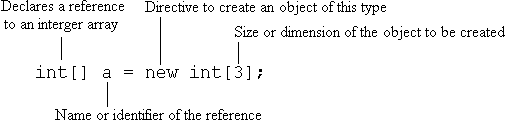This creates an array of integers as follows: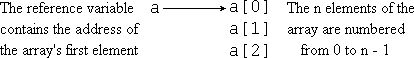A different size of interger array object can be assigned to the array reference 'a' at any time as follows:

a = new int;

Java's built-in automatic garbage collection service gets rid of the old de-refer­enced 3-element array.

The size or length of the object currently referenced by reference variable 'a' is always available in the length variable a.length. This is illustrated in the program below which prints out the contents of the array.

for(int i = 0; i < a.length; i++)
System.out.println(i + ": " + a[i]);

Arrays of Objects

Arrays can contain objects other than Java's primitive types like int, float etc. For instance, you can create an array of objects of a class called X as follows:

X[] a = new X;

Suppose that X is a name and address class containing a person's name, job title, company, premises name, street name, town, county, postcode. Now suppose that Class Y extends X to define a Contact Record class which includes all of the above plus phone and fax numbers, Email address and Web Site. You can create an array of objects of Class Y as follows:

Y[] b = new Y;

Each element of b[] contains everything that each element of a[] contains and more. The X-fields of each element of an array of Y objects can therefore be pro­cessed by any method() which has been designed to process arrays of pure X objects. Therefore a reference to a Y object can be used anywhere that a reference to an X object can be used.

The sense in which an array is an object is limited in that an array cannot itself be extended. You can only extend objects which can later become elements of an array of the extended objects. For example, to create an array of actual objects of type X, you have to proceed as follows:

X[] x = new X;  /*create a 12-element array of pointers to
objects of Class X. The elements of this
array initially contain null pointers. */

for(int i = 0; i < x.length; i++)
x[i] = new X(names[i], jobtits[i], ... );

Each pass of the for loop creates an object of Class X which becomes an element of the array x[]. During this process, the address of each new object is put in the ith element of the array of references (pointers) x[].

Arrays of Arrays of Objects

Only single-dimensional arrays of primitive types or objects exist in Java. Multi-dimensional arrays are effected by creating arrays of pointers to sets of single-dimensional arrays. For example, to declare a 4 x 5 array of float variables, m, you write:

float[][] m = new float[];
for(int i = 0; i < m.length; i++)
m[i] = new float;

The first statement generates a 4-element array of pointers to floats whose ele­ments are all initialised to null. Each pass of the for loop then generates a 5-element array of floats, the start address of which is placed in the appropriate element of the pointer array created earlier. The structure thus generated is shown below: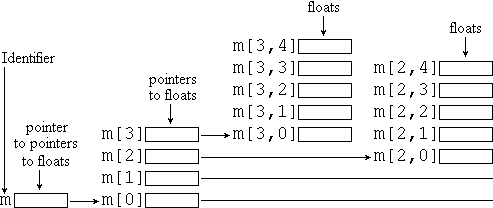It is not necessary that all the arrays of floats be the same length. You can for example create a 2-dimensional array of objects whose rows are of different lengths. To print out the elements of the above array write:

for(int i = 0; i < m.length; i++) {
for(int j = 0; j < m[i].length; j++)
System.out.print(m[i][j] + " ");
System.out.println();
}

How to Initialise Variables

A variable can be initialised in its declaration statement: eg.

final double p = 3.14159d
float r = 1.0f

Variables which are fields of a class are automatically initialised to default values as follows:

boolean: false 'u0000' 0 +0.0f, +0.0d null

However, Java doesn't initialise the local variables in a block to the above defaults. You must initialise them explicitly. Local variables are initialised each time their declarations are executed. Object fields and array elements are initialised when they are created with a new. All static variables within the bounds of a class are initialised before any code in that class is run.

To declare and initialise a string array you can write for example:

String[] d = new String;
d = "Lions";
d = "Tigers";
d = "Bears";

But this can be short-handed to:

String[] d = {"Lions", "Tigers", "Bears"};

To initialise a 4 x 4 matrix of doubles using this short-hand form, write:

double[][] m = {
{1.0, 0.0, 0.0, 0.0},
{0.0, 1.0, 0.0, 0.0},
{0.0, 0.0, 1.0, 0.0},
{0.0, 0.0, 0.0, 1.0}
};

Java's Operator Precedence

Uniary Operators

 postfix operators: x[]     x.     (x,y,z)     x++     x−− prefix operators: ++x     −−x     +x     −x     ~x [bitwise NOT]     !x creation operator: new x cast operator: (type)x

Binary Operators [these are left-associative]

multiplicative operators: *   /   % +   − <<   >>   >>> [unsigned right-shift] <   >   >=    <=   instanceof ==   != & ^ | && || ?   :

Associative Operators [these are right-associative]

=    +=     −=     *=    /=     %=     >>=     <<=     &=     ^=     |=

Use parentheses to override precedence where necessary eg:

while((v = stream.next()) != null)
process(v);

Java Expressions

Expressions are evaluated strictly left-to-right. For example, x + y + z is eval­uated in the following order.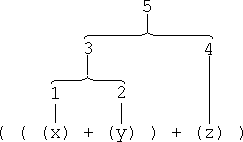This order of evaluation is guaranteed. This can matter when the operands are re­sults returned by embedded function calls.

The type of the result of an expression evaluation is determined as follows:

int   +  int    =  int
int   +  long   =  long

float +  int    =  float
float +  long   =  float

float +  float  =  float
float +  double =  double

where + represents any arithmetic or bit-wise operator.

string  +   any type  =  string
string  +=  any type  =  string

Any interger type less than 32 bits long is promoted to a 32-bit int before it takes part in the evaluation of an expression as follows:

byte:     top 24 bits filled with the value of the sign bit
short:    top 16 bits filled with the value of the sign bit
char:     top 16 bits set to zero

Java Type Conversions

Implicit Conversions

int  x = 5;      //x is a 32-bit interger
int  y = 40;     //y is another 32-bit interger
long z = x + y;  //z is a 64-bit interger

Implicit type conversions take place automatically in the third line above as illust­rated below:

long z = (long)x + (long)y;

float x = 29.5;     //x is a 32-bit IEEE floating point value
float y = .00037;   //y is a 32-bit IEEE floating point value
double z = x + y;   //z is a 64-bit IEEE floating point value

Implicit type conversions take place automatically in the third line above as illust­rated below:

double z = (double)x + (double)y;

long x = 0x7effffffffffffffL;   //x has 64-bit precision
float y = x;                    /* but y's mantissa has only
24-bit precision */
y can accommodate the range, but not the precision of x. Some of x's 64-bit precision therefore is lost when its value is assigned to y.

Explicit Conversions

double x = 7.99;      //48-bit mantissa + 16-bit exponent
long   y = (long)x;   //cast to a 64-bit interger

Although it can accommodate greater precision, y holds only the truncated value of x, namely 7. That is why this conversion is not automatically allowed, but must be explicitly written into the code.

double x = BigNum;        /* double has a greater range
and precision than float */
float y = (float)x;       /* so this conversion must be
forced explicitly */

Can result in a serious loss of both range and precision if you aren't careful.

long x = 0x0f0fffff;  //a long whose lower 16 bits are all set
char y = (char)x;     //lower 16-bits only used so y = \uffff
short c = (short)y;   //same length but the value in c = -1
int z = (int)y;       //top 16 bits filled with zeros z = 65535

Reference Conversions

An object reference (what most of us know as a pointer) can be converted to refer to different types of object.

Obgekt p = null;
ExtendedObgekt q = p;	//this assignment conversion is implicit

ExtendedObgekt p = null;
Obgekt q = (Obgekt)p;	//but this conversion must be explicit

However, the above can be forced by explicitly typecasting the extended object as an object, for example:

teeshirt p = TeeShirts[x];
clothing q = (clothing)p;

This is valid when you want to perform a function on a teeshirt object which could be equally well performed on an item of clothing of any kind, such as reading its washing label viz:

where the above is a method within the Class Clothing{ } of which the Class TeeShirt{ } is an extension.

To test whether teeshirt is an extension of clothing, thus avoiding a possible error use the test:

if(teeshirt instanceof clothing)
q = (clothing)p;

String Conversions

long x = 23.789;
string y = "Output Value = " + x;

This is interpreted as:

string y = "Output Value = " + toString(long x);

A toString() method is defined for all primitive types and one can be written into the class code for any object.

How to Access Members of a Class

Members (or elements) of an array are accessed by using the [] operator. The fol­lowing accesses the ith member of the interger array X:

int x = X[i];

Members (fields) of objects are accessed using the . operator as follows:

int lat = NavStn.lat; //access latitude of a navigation station
int lng = NavStn.lng; //access its longitude also

where NavStn is an object reference pointing to an instance of the class of objects called NavStns{ }.

Members of the class itself — i.e. fields which pertain to the class as a whole rather than to individual objects of that class — are accessed in the same way. But, this time you use the class name instead of an object reference:

int NumStns = NavStns.NumStns; /*access the number of navigation
stations currently on file */

Methods are also members of classes. Consequently they are accessed in the same way. The following example finds a station's current bearing:

int brg = NavStns.GetBrg(lat, lng);

Exceptions: If you access an object or array with a reference whose value hap­pens to be null, then a NullPointerException is thrown. If you specify an array element number which is out of range (e.g. you ask for the 23rd element of an array which has only 16 elements), then an IndexOutOfBoundException is thrown. Java omits this check if at compile time it can be determined that the index cannot ever go out of range (e.g. in a loop of defined limits).

A chocolate cake is a specific kind of cake. A cake is a specific kind of dessert. A buttered scone is a specific kind of scone. A scone is a specific kind of dessert.

Suppose we have three methods all with the same name and each with the same number of parameters. However, the parameters are of different object types:

1. void arrange(Dessert d, Scone s);
2. void arrange(Cake c, Dessert d);
3. void arrange(ChocolateCake cc, Scone s);

Which version of the method will the following statements invoke?

arrange(dessertRef, sconeRef);

It invokes No 1 because the parameter types are an exact match.

arrange(chocolateCakeRef, dessertRef);

This invokes No 3 because chocolate cake is a more specific form of cake and scone is a more specific kind of dessert.

arrange(chocolateCakeRef, butteredSconeRef);

This invokes No 3 because chocolate is an exact match and buttered scone is a more specific type of scone.

arrange(cakeRef, sconeRef);

This would tend to invoke Nos 1 and 2 equally and therefore cannot invoke either. It is an invalid invocation.

The same rules apply to primitive types. E.g. an int is assignable to a float just as a buttered scone is assignable to a scone. But if you declare two methods which both compute the distance to a navigation station:

int dist(int lat, int lng){   }
short dist(int lat, int lng){   }

and then you invoke one of them by the statement:

double d = dist(lat, lng);

the compiler has no way of knowing which one to invoke here — even if the two methods throw different exceptions.

The moral is that when in doubt use the method's complete class reference.

Java Integer Arithmetic

 0111 +7 0110 +6 0101 +5 0100 +4 0011 +3 0010 +2 0001 +1 0000 +0 1111 −1 1110 −2 1101 −3 1100 −4 1011 −5 1010 −6 1001 −7 1000 −8
JAVA interger arithmetic (int and long) cannot overflow or underflow: it simply wraps round and comes full circle.

The figures at the left illustrate the principle of two's complement arithmetic on which all interger arithmetic in JAVA is based.

Notice how the bits change around zero. Minus 1 is all bits set, zero is no bits set, plus 1 is the least significant bit set. Notice too that two's complement arithmetic wraps round from + 7 to −8! One more num­erically.

The full range of values is shown for only a 4-bit two's complement register so that the full range can be illustrated in half a page.

To convert a negative number to its positive equivalent and vice versa, invert all the bits then add 1.

Java's int is a 32-bit register. Its critical wrapping at zero and at its numerical maxima are shown below.

Around zero it behaves as follows:

00000000000000000000000000000010	+2
00000000000000000000000000000001	+1
00000000000000000000000000000000	+0
11111111111111111111111111111111	-1
11111111111111111111111111111110	-2
Around its maximum values it behaves as follows:
10000000000000000000000000000010	-4294967294
10000000000000000000000000000001	-4294967295
10000000000000000000000000000000	-4294967296
01111111111111111111111111111111	+4294967295
01111111111111111111111111111110	+4294967294

A short (16-bit), int (32-bit), and long (64-bit) arithmetic all follow the same rules. Arithmetic performed on a char (16-bit Unicode) converts implicitly to int before applying the actual arithmetic operations.

The arithmetic operations which can be performed on integers are:

 x + y add x to y (always wraps) x - y subtract y from x x * y multiply x by y x / y divide x by y x % y gives the remainder when x is divided by y

The action of the remainder operator is to repeatedly subtract y from x until it can­not get a complete y. It then returns what is left. Eg: 15 % 4 works out as:

15 - 4 = 11
11 - 4 =  7
7 - 4 =  3
3 - 4 cannot go so it returns 3 as the answer.

The remainder function is defined formally by the identity:

( x / y ) * y + x % y == x

Interger multiplication wraps. Interger division truncates towards zero: eg:

+7 / 2 = +3
and -7 / 2 = -3

x / 0 and x % 0 are invalid and throw an ArithmeticException. Java arithmetic also supports the 'minus' unary operator to negate the value to which it is applied e.g. -3

For symmetry it also supports the unary 'plus' operator e.g. +3

Java Floating-Point Arithmetic

An IEEE 754-1985 subset:

Underflows to zero: Overflows to infinity: +0−0 if decrementing to zero from the positive side if incrementing to zero from the negative side +∞−∞ if incrementing upwards to infinity if decrementing downwards to minus-infinity

You can initialise a variable to infinity for example:

float x = float.NEGATIVE_INFINITY;
initialises the IEEE 32-bit floating-point variable x to minus-infinity.

A Java floating-point variable can also hold a value called NaN which stands for 'not a number'. It results when the outcome of a floating-point arithmetic operation is what mathematicians call 'indeterminate'. The behaviour of Java's floating-point arith­metic in operations involving signed zeros and infinities is illustrated by the following identities:

+∞ + +∞ ≡ ∞ +∞ − +∞ ≡ NaN −∞ − −∞ ≡ NaN +∞ − −∞ ≡ ∞ x % 0 ≡ NaN x % ±∞ ≡ x 0 % 0 ≡ NaN ±∞ % x ≡ NaN ±∞ % ±∞ ≡ NaN

Java floating-point arithmetic does not throw exceptions like invalid operations, divi­sion by zero, overflow, underflow or inexact. Nor does it signal the occurrence of a NaN result. It just carries on by rounding results of operations towards zero or the nearest representable value, preferring values with the least-significant bit set to zero.

Also, you cannot use things like <= or >= in Java floating point. E.g. you must use if(!(x < y)) instead of if(x >= y).

Java's ++ and -- Operators

The statements i++; and ++i; both increase the value of i by 1.
The statements i--; and --i; both decrease the value of i by 1.

Therefore both i++; and ++i; are equivalent to i = i + 1;
and both i--; and --i; are equivalent to i = i - 1;

However there is a difference:
x = a[++i]; is equivalent to i = i + 1; x = a[i];
x = a[i++];
is equivalent to x = a[i]; i = i + 1;

If instead of incrementing the index of an array, we increment the contents of one of its elements, then both a[i]++; and ++a[i] are equivalent to a[i] = a[i] + 1; and a[i]--; and --a[i] are equivalent to a[i] = a[i] - 1;

However, if i is obtained from a method m( ) which returns a different value each time it is called as in a[m(i)]++; , then a[m(i)]++; is not equivalent to a[m(i)] = a[m(i)] + 1; and a[m(i)]--; is not equivalent to a[m(i)] = a[m(i)] - 1;

The statement a[m(i)]++; increments a particular element of a[] while the state­ment a[m(i)] = a[m(i)] + 1; sets the value of one element of a[] to one more than the value of some other element of a[].

Illustration of the effects of ++i and i++:

class IncOrder {
public static void main( String[] args ) {
int i = 16;
System.out.print(++i + " " + i++ + " " + i);
}
}

Output is 17 17 18.

Java's Relational Operators

These operators return a boolean result true or false.

> greater than less than greater than or equal to less than or equal to equal to not equal to

Only the last two can be used in expressions relating boolean variables. The prefix operator ! means 'not'. It inverts the boolean result of what immediately follows it.

These operators return a boolean value indicating whether a particular relationship between two variables is true or false.

For example, in the test: if(x < y) Java evaluates the expression x < y as a behind-the-scenes boolean variable b whose value is either true or false. It then uses the value of b in the implied statement if(b == true) to determine which route to take.

Test boolean values directly:

If x and y are boolean variables, use a test of the form: if(x || !y ){ ... }
instead of: if(x == true || y == false){ ... }

Single expressions which evaluate to a boolean value can be joined to form a com­posite expression using: && (conditional AND) and || (conditional OR). For example:

if( x && y ) { ... }

Java evaluates as little as it has to in order to obtain a valid result. For instance, in

if(i >= 0 && i < a.length && a[i] != 0){ ... }

if i is negative, the other two expressions are not evaluated.

Because == and != can only relate boolean values, they can be used to construct an exclusive OR or XOR test as follows:

if(x < 0 == y < 0)
SameSign();
else
DiffSign();

x != NaN always returns true
x # NaN always returns false where # is any relational operator other than !=.

If s1 and s2 are string pointers, the expression s1 == s2 is true only if s1 and s2 point to the same string array: it does not test to see if two different string arrays have the same content.

Java's Bitwise Operators

Logical Operators

& bitwise AND bitwise OR bitwise exclusive OR ie XOR bitwise inverter, eg ~1101 becomes 0010

Shift Operators

<< Shift left (shifting zero bits in from the right) Shift right (shifting with sign bits in from the left) Shift right (shifting zero bits in from the left)

 1101 1101 1101 << 2 becomes 0100 >> 2 becomes 1111 >>> 2 becomes 0011

The number of bit-places shifted (the right operand) is the number you provide masked by (the size of the type of the left-hand operand) - 1. So if the left-hand operand is a 32-bit int, the mask is 31

 so a shift of 00000000000000000000000000100011 11111111111111111111111111100011 00000000000000000000000000011111 00000000000000000000000000000011

Bitwise operators & and | can be used on boolean variables in place of && and ||. However unlike && and ||, & and | always evaluate both operands before returning their result — so beware.

A converse logical XOR test can be constructed using the bitwise XOR operator ^ which returns a true if the expressions evaluate to opposite boolean states and false if to the same boolean state as in:

if((x < 0)^(y < 0))
DiffSign();
else
SameSign();

Java's Conditional Operator ? :

value = UserSetIt ? UsersValue : DefaultValue;

is equivalent to:

if(UserSetIt)
value = UsersValue;
else
value = DefaultValue;
Consider the types of the variables involved.
double scale = halveIt ? 1 : 0.5;

The types of the second and third operands must be the same as, or assignable to, the type of the value (i.e. the left side of the = sign).

Java's Assignment Operators

The most basic assignment operator is '='.

However, in Java, any arithmetic or bitwise operator can be joined to '=' to form a composite assignment operator. For example:

a[func()] += 1; is equivalent to a[func()] = a[func()] + 1;

However, in the first case, func() is called only once. Since it may return a new value when called a second time, we cannot be sure whether or not it is the same array element that is being referred on both the left and right hand sides of the '=' sign. The composite '+=' operator is therefore clearer and more predictable in operation when a function is used as an array indexer.

If var is a variable of type Type, then

var op= expr is equivalent to var = (Type)((var) op (expr))

Note that the whole of expr is bound tightly. For example:

a *= b + 1; is equivalent to a = a * (b + 1);
not a = a * b + 1;

Although a += 1; is the same as ++a; the ++ is traditionally the preferred coding.

Java's Flow Controls

 A statement: assignment; A block: {assignment; assignment; ... }

if(boolean condition)
statement; or {block}
else  //an 'else' is always associated with the most recent 'if'
statement; or {block}

switch x {
case A:  statement; or {block}          //fall through
case B:  statement; or {block}          //fall through
case C:  statement; or {block} break;   //skip default
default: statement; or {block}
}

while(condition) statement; or {block}

do statement; or {block} while(condition)

for(initializers; condition; incrementers)
statement; or {block}

label: statement; or {block}

Bailing Out

break; exit the inner-most block in which it occurs exit the labelled block called 'label' immediately start the next pass of the inner-most loop immediately start the next pass of the labelled loop return from the current method to its caller return from the current method, passing the value of x to its caller. The 'type' of 'x' must be the return type declared for this method.

NOTE: There's no 'goto' statement in Java because 'break' and 'continue' can ex­press more formally what 'goto' is used for in other languages.